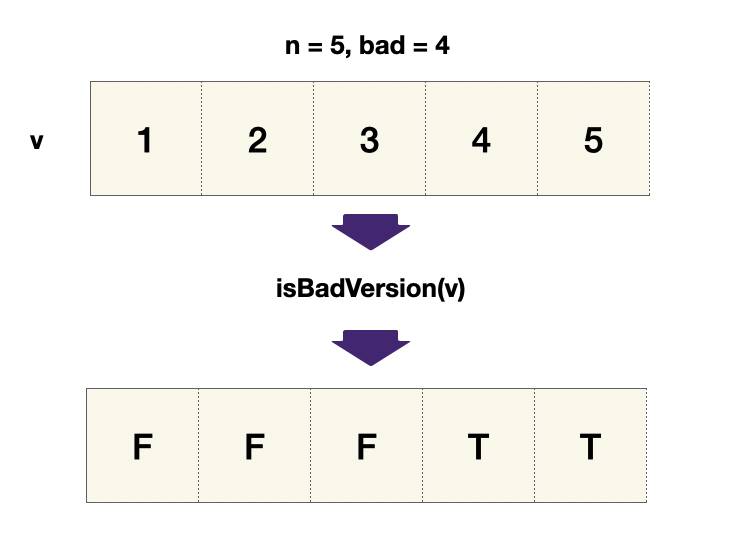You are a product manager and currently leading a team to develop a new product. Unfortunately, the latest version of your product fails the quality check. Since each version is developed based on the previous version, all the versions after a bad version are also bad.

Suppose you have `n` versions `[1, 2, ..., n]` and you want to find out the first bad one, which causes all the following ones to be bad.

You are given an API `bool isBadVersion(version)` which returns whether `version` is bad. Implement a function to find the first bad version. You should minimize the number of calls to the API.

Example 1:
Input: n = 5, bad = 4
Output: 4
Explanation:
Then 4 is the first bad version.

Example 2:
Input: n = 1, bad = 1
Output: 1\

Constraints: `1 <= bad <= n <= 231 - 1`

## Solution

Intuitively, we know that the product versions is a sequence of good versions, followed by a group of bad versions. This means that we are looking for the first index which `isBadVersion(ans)` returns `true`. Therefore, we must update the answer when we find a smaller version that is bad, repeat until we find the smallest.``````1def firstBadVersion(self, n: int) -> int:
2    left, right = 1, n
3    ans = -1
4
5    while left <= right:
6        mid = (left + right) // 2